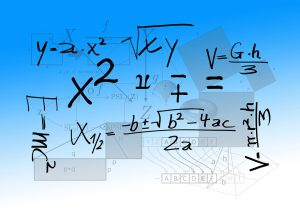# Basic Operations Exercises

October 23, 2016
By

Want to share your content on R-bloggers? click here if you have a blog, or here if you don't.This set of exercises will help you to learn and test your skill with basic arithmetical operations and logic functions. Before proceeding, it might be helpful to look over the help pages for the `**`, `%/%`, `%%`, and the logical operators such as `!=, ==, >=, isTRUE `.

Answers to the exercises are available here.

If you obtained a different (correct) answer than those listed on the solutions page, please feel free to post your answer as a comment on that page.

Exercise 1

Basic Operations There are two main different type of interest, simple and compound. To start let’s create 3 variables, S = 100 (initial investment), i1=.02 (annual simple interest), i2=.015 (annual compound interest), n=2 (years that the investment will last).

Simple Interest Define a variable called `simple` equal to S (1 + i1 * n)

Compound Interest Define a variable called `compound` equal to S x (1 + i2)n

Exercise 2

It’s natural to ask which type of interest for this values gives more amount of money after 2 years (n = 2). Using logical functions ` <,>, == ` check which variable is bigger between `simple` and `compound`

Exercise 3

Using logical functions ` <,>, ==, |, & ` find out if simple or compound is equal to 120

Using logical functions ` <,>, ==, |, & ` find out if simple and compound is equal to 120

Exercise 4

Formulas can deal with vectors, so let’s define a vector and use it in one of the formulas we defined earlier. Let’s define S as a vector with the following values 100, 96. Remember that `c()` is the function that allow us to define vectors.

Apply to S the simple interest formula and store the value of the vector in `simple`

Exercise 5

Using logical functions ` <,>, == ` check if any of the `simple` values is smaller or equal to `compound`

Exercise 6

Using the function `%/%` find out how many \$20 candies can you buy with the money stored in `compound`

Exercise 7

Using the function `%%` find out how much money is left after buying the candies.

Exercise 8

Let’s create two new variables, ode defined as `rational=1/3` and `decimal=0.33`. Using the logical function `!=`
Verify if this two values are different.

Exercise 9

There are other functions that can help us compare two variables.

Use the logical function `==` verify if `rational` and `decimal` are the same.

Use the logical function `isTRUE` verify if `rational` and `decimal` are the same.

Use the logical function `identical` verify if `rational` and `decimal` are the same.

Exercise 10

Using the help of the logical functions of the previous exercise find the approximation that R uses for 1/3. Hint: It is not the value that R prints when you define 1/3

R-bloggers.com offers daily e-mail updates about R news and tutorials about learning R and many other topics. Click here if you're looking to post or find an R/data-science job.
Want to share your content on R-bloggers? click here if you have a blog, or here if you don't.